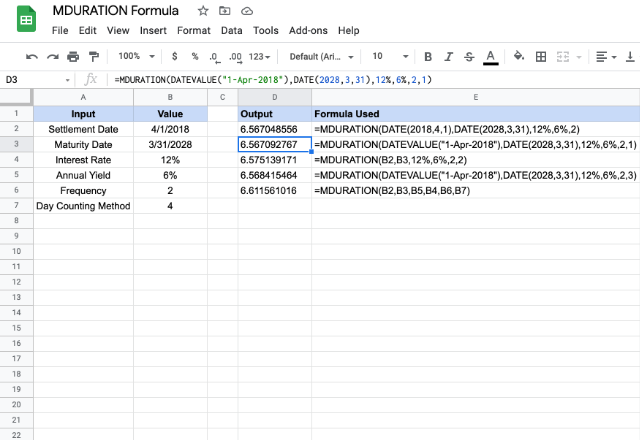# How to use the MDURATION formula in Google SheetsGiven the expected yield that an investment and the interest rate, the MDURATION formula in Google Sheets calculates the modified Macaulay duration. It is the weighted average before the buyer would receive the investment’s cash flows. Also, the formula will require other inputs as explained below, for it to be able to evaluate the duration correctly.

### Syntax

MDURATION(settlement, maturity, rate, yield, frequency, [day_count_convention])

• settlement – is the date after issuing the security, when it is actually delivered to the buyer.
• maturity – is the end or maturity date of the security, when the buyer can redeem it at face or par value.
• rate – is the annualized interest rate at which the investment appreciates.
• yield- is the annual yield that the buyer expects for the security.
• frequency – is the number of interest payments that buyer can pay per year.
• day_count_convention – [OPTIONAL parameter – 0 by default] – it is an indicator of the day count method that Google Sheets should consider. There are five different possible values for this parameter.
• 0 – assumes that there are 30 day months and 360 day years (US (NASD) 30/360). Using this value ensures there are specific adjustments to the entered dates that usually are at the end of months.
• 1 – calculates based on the actual number of days between the specified dates, and the actual number of days in the intervening years.
• 2 – calculates based on the actual number of days between the specified dates, but assumes a 360 day year.
• 3 – evaluates the DURATION formula in Google Sheets based on the actual number of days between the specified dates, but assumes a 365 day year.
• 4 – very similar to the first option 0, except, it adjusts end-of-month dates according to European financial conventions.

### Usage: MDURATION formula in Google Sheets

Seemingly the formula feeds on multiple parameters and it might look a little complex. But understanding the concepts with the help of a few examples should make us feel at ease. So, let’s dive into them head first.Please note that the settlement and maturity parameters require valid dates. Therefore, we need to use either the results from formulas such as DATETO_DATE, or references to other date type cells. If we do not ensure this, Google Sheets may return parsing errors. Similarly, the other parameters – rateyieldfrequency, and day_count_convention – can be direct numeric values or references to the cells holding the appropriate values.

We might notice that all the formulas seem to produce different output values. Even if the initial parameters effectively being the same. This is happening due to the day counting method which we indicated using the final day_count_convention parameter.

### MDURATION formula

And there you go! Use the MDURATION formula in Google Sheets to calculate the modified Macaulay duration.

If you’d like to learn more about the various formulas of Google Sheets, why not take a look at our blog post on the DURATION formula in Google Sheets.

Alternatively, check out related blog posts below!